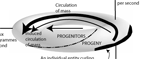﻿ Four maxims of ecology | Engenetics: The science of species

# The four maxims of ecology

## The bioactivity of a biological population is subject to increase from an initial value for one or more of three reasons: (a) increases in mass; (b) decreases in competition. All other increases are due to (c) the essential development of the entity or species.

##### Explanation

G  O    T  O    C  O  N  S  T  R  A  I  N  T  SLAW 1: The law of existence
n ≥ 1; δW = (δQ - dU) > 0; m → ∞; > 0

LAW 2: The law of equivalence
[(δW1 = δW2) ∧ (δW2 = δW3)] ⇒ (δW1 = δW3)

LAW 3: The law of diversity
A → 0; FM

LAW 4: The law of reproduction
[(dm̅/dt ≤ 0) ∧ ( > 0)] ⇒ [(dn/dt ≥ 0) ∧ (dA/dt > 0)]

dU = Mdt = δQ - dH; M > 0
Biology is “the study of those systems that can replace their internal energy” (See explanation of terms and variables).

pdt + mdt = dh + du = δq; m > 0
Ecology is “the study of the processes systems use to replace their internal energy” (See explanation of terms and variables).

The Gibbs-Duhem equation governs all biological energy:
m̅μ = dS = dU + dH - Σi μi(dvi - dmi)
The Euler equation governs all biological activity:
μ = dS = (∂S/∂U)V,Ni dU + (∂S/∂V)U,Ni dV + Σi (∂S/∂ui)U,V,{Nj≠i} dui + Σi (∂S/∂vi)U,V,{Nj≠i} dvi.

All biological populations are subject to the three constraints of:

(a) constant propagation, ;
(b) constant size, ;
(c) constant equivalence, .